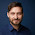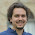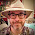### Animating Random Projections of High Dimensional Data

Recently Jake showed some pretty cool videos in his blog.
This inspired me to go back to an idea I had some time ago, about visualizing high-dimensional data via random projections.

I love to do exploratory data analysis with scikit-learn, using the manifold, decomposition and clustering module. But in the end, I can only look at two (or three) dimensions. And I really like to see what I am doing.

So I go and look at the first two PCA directions, than at the first and third, than at the second and third... and so on. That is a bit tedious and looking at more would be great. For example using time.

There is a software out there, called ggobi, which does a pretty good job at visualizing  high dimensional data sets. It is possible to take interactive tours of your high dimensions, set projection angles and whatnot. It has a UI and tons of settings.
I used it a couple of times and I really like it. But it doesn't really fit into my usual work flow. It  has good R integration, but not Python integration that I know of. And it also seems a bit overkill for "just looking around a bit".

So I thought I could try something in the same spirit, but way simpler.
I'll have a look at there work again and see what kind of smart things they came up with, but for now, I'll just follow my nose and do something stupid.

So let's start with iris. This is the most simple data set ever, with three classes, four dimensions, and most of the variation in the first two PCA directions.
You can get a pretty good idea by looking at the first three PCA directions (colors code classes):

But we wanted to look at all four. To do this, I want a projection that is parametrized, so I can vary it over time. Basically I want a 1d trip (the time) through the space of all 2d projections of iris.
It's not so easy to cover all, so lets just start with some tour that get's around.
Starting from a PCA'ed version of iris in X_pca, we take

```interpolation1 = np.cos(alpha) * X_pca[:, 1] + np.sin(alpha) * X_pca[:, 2]
interpolation2 = np.cos(beta) * X_pca[:, 0] + np.sin(beta) * X_pca[:, 3]
```

So we take some angle alpha between the second and third direction, and some angle beta between the first and fourth.
As time only has one dimension, I have to give alpha and beta a common parametrization. I chose beta to go twice as fast as alpha.

Using this together with matplotlib.animation.FuncAnimation gives (drums)

I think that looks pretty neat already. (Apart from bad video quality :-/ sorry for that. I blame blogger.)
The full code is here and just a couple of lines.

Now let's move to a bit more high-dimensional data.
I went with the digits dataset in scikit-learn, which is 64 dimensional, which is kind of hard to look at already. To be able to see something, I just used the classes 1, 2 and 7 (otherwise it gets pretty crowded).
The tour I used for iris was pretty arbitrary and now I wanted something that would work more or less with an arbitrary number of dimensions.

What I ended up doing is use a projection matrix and vary each entry with a slightly shifted sine-wave. This gives a rotating smooth look and also makes a close loop, so you can repeat the video.
Here is what the result of using PCA on the three classes of digits looks like:
Looks pretty good already (this data set is also very easy), but it is not so clear whether 1 and 2 can be easily linearly separated.
So let's have a look at the video:

And yes, indeed: If you watch the video a bit, it's easy to convince yourself that all classes are pretty well linearly separable.

The code that does the animation is this piece:

```def animate(self, i):
# set top 2x2 to identity
self.projection[0, 0] = 1
self.projection[1, 1] = 1
# set "free entries" of projection matrix
# gives them a "rotation" feel and makes the whole thing seamless.
scale = 2 * np.pi * i / self.frames
self.projection[2:, :] = np.sin(self.frequencies * scale + self.phases)
interpolation = np.dot(X, self.projection)
# normalize so we fit on screen
interpolation /= interpolation.max(axis=0)
for p, c in zip(self.points, np.unique(y)):
p.set_data(interpolation[y == c, 0], interpolation[y == c, 1])
return self.points
```

The full code is here. It should be generic and I invite you to play with it :)
If you wonder about the 2x2 identity that I used, let me go off on a slight tangent...
(if you're not into math, skip this ;)

As I said before, we want to have a tour of the 2d projections of our data space.
The set of 2d projections is given by the Grassmannian manifold - usually this is thought of as the space of all subspaces, but we can also think of it as the space of all projections.
A point on the Grassmannian can be represented by the basis of the subspace we are interested in. But many different basis can give rise to the same subspace, i.e. all linear combinations yield the same subspace. We can get rid of that redundancy by fixing a square sub-part (for example the first two rows as I did above) and demand that this part is the unit matrix.
Not all subspaces can be expressed in this way - for example the one that has only zeros where I want the unit matrix. But the set is dense in the Grassmannian, which basically means I get "nearly all" points.

I doubt this makes any difference to the video but I like to think my math education (pdf) was not totally wasted ;)

Enjoy and I'd love to hear any feedback :)

1.You should really check out Ggobi (www.ggobi.org)

2.3.4.Thanks for your write up. I look forward to playing with the code this week.